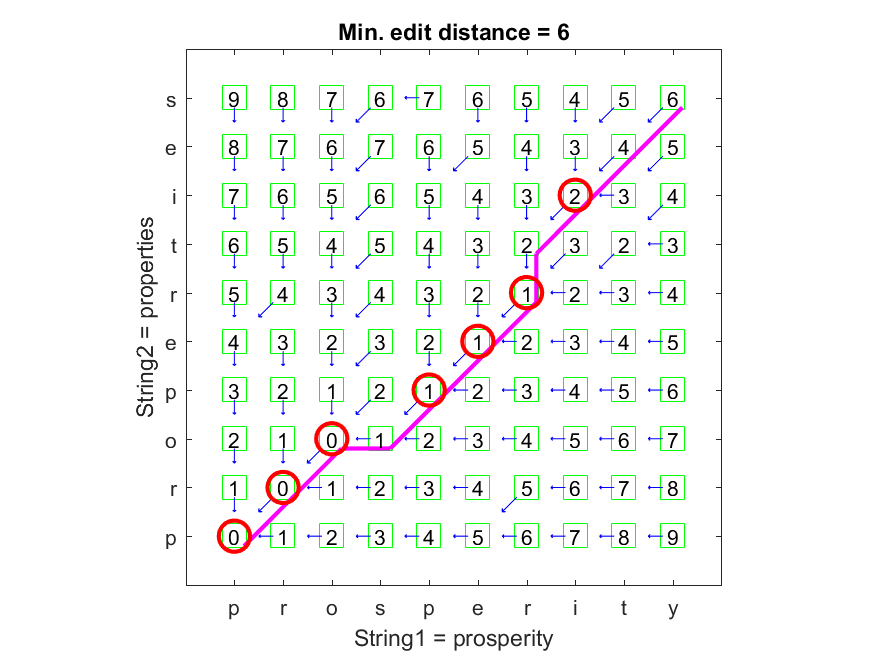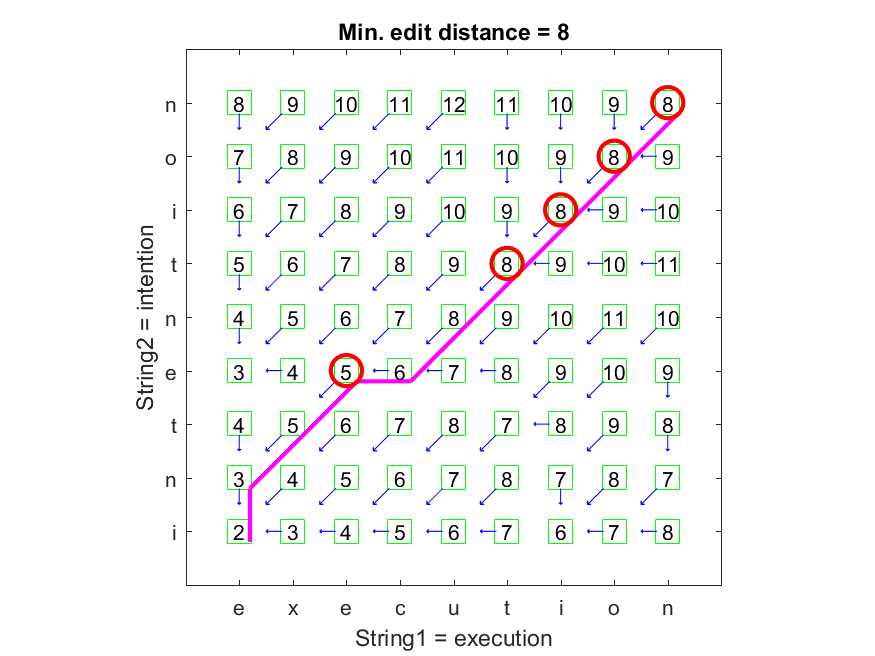8-3 Edit Distance

[chinese][all]

We can use three basic operations of "delete", "insert", and "substitute" to convert a string into another one. The edit distance between two strings is defined as the minimum number of the basic operations that are required to converting a string into another. Similarly, we can also define the costs of these operations and then find the required operations for conversion one string to another with the minimum cost.

We can also use the concept of DP to compute the edit distance between two strings. Let the optimum-value function ED(a, b) defined as the edit distance between strings a and b. Then the recursive formula for ED is shown next, assuming the costs of "delete" and "insert" are 1, while "substitute" is 2.

1. ED(ax, by) = ED(a, b) if x = y.
2. ED(ax, by) = min(ED(a, b)+2, ED(ax, b)+1, ED(a, by)+1) if x ≠ y.
The boundary conditions are ED(a, []) = len(a), ED([], b) = len(b).

Hint
The edit distance is also used in the command "fc" (under DOS) or "diff" (under UNIX) for comparing the contents of two text files.

The following example demonstrates a typical result of edit distance of strings "prosperity" and "properties":

Example 1: editDistance01.mstr1 = 'prosperity'; str2 = 'properties'; substituteCost=2; plotOpt = 1; [minDist, edPath, edTable] = editDistance(str1, str2, substituteCost, plotOpt);As shown in the plot, the circle positions indicate common characters.

The next example demonstrates the result of edit distance between "execution" and "intention", which is the example used in 3.11 of "Speech and Language Processing".

Example 2: editDistance02.mstr1 = 'execution'; str2 = 'intention'; substituteCost=2; plotOpt = 1; [minDist, edPath, edTable] = editDistance(str1, str2, substituteCost, plotOpt);Data Clustering and Pattern Recognition (資料分群與樣式辨認)